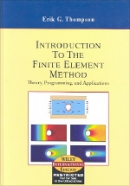## 도서정보기계/재료/화공 원서Introduction to the Finite Element Method 2/E 저자 / Erik G. Thompson 저 가격₩- 출간일2004.01.01 ISBN 100471267538 페이지360 차례 1. Introductory Example 2.Calculus of Variations 3. Program Wire 4. The FEM for Second Order ODE'S 5. A Finite Element Function for Two Dimensions 6. Poisson's Equation—FEM Approximation 7. Poisson's Equation—Applications 8. Higher Order Elements 책소개 The right balance of theory, programming, and applications Erik Thompson presents the theory, applications, and programming skills you'll need to understand the finite element method and use it to solve problems in engineering analysis and design. Offering concise, highly practical coverage, this introductory text presents complete finite element codes that can be run on the student version of MATLAB or easily converted to other languages. Master the basic theory: The text promotes an understanding and appreciation of the theoretical basis of finite element approximations by building on concepts that are intuitive. Throughout, the text uses matrix notation to help you visualize the finite element matrices. Study problems reinforce basic theory. Experiment with the code: Numerical experiments show how to test programs for possible errors, experiment with boundary conditions, and study accuracy and stability. Code development exercises suggest ways to modify the codes to create additional capabilities. All codes are available on the book's web page along with sample data files for testing them. Each code can be immediately run using only the student version of MATLAB. Because each code is written using explicit programming, they also serve as pseudo-codes that can be used to develop programs in any computer language. Gain hands-on experience: Projects, representing a wide variety of engineering disciplines, enable you to conduct analyses of fairly complex problems. Many of these projects encourage you to investigate new techniques for using the finite element method. 저자소개 Erik G. Thompson 저